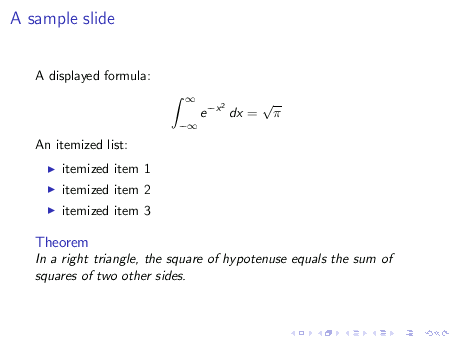# 3  A bare-bones sample

The basic structure of a Beamer input file is pretty simple. Consider the file small.tex, the content of which is shown below:

% small.tex
\documentclass{beamer}
\usetheme{default}
\begin{document}

\begin{frame}{A sample slide}

A displayed formula:

$\int_{-\infty}^\infty e^{-x^2} \, dx = \sqrt{\pi}$

An itemized list:

\begin{itemize}
\item itemized item 1
\item itemized item 2
\item itemized item 3
\end{itemize}

\begin{theorem}
In a right triangle, the square of hypotenuse equals
the sum of squares of two other sides.
\end{theorem}

\end{frame}

\end{document}


The input file above produces the following slide:Remark: In Beamer, a frame is what you would normally call a “slide”. The image shown above is the realization of a single “frame”. In what follows, I use the terms “frame” and “slide” interchangeably.1

The \begin{frame}...\end{frame} block may be repeated any number of times to produce a sequence of slides.

Remark: In the example above we have:

\begin{frame}{A sample slide}
...
\end{frame}


The “A sample slide” is the slide’s title. Its use is optional but it is only in very rare cases where omitting it would make sense.

Beamer provides an alternative method for specifying a slide’s title. The following is exactly equivalent to the fragment shown above:

\begin{frame}
\frametitle{A sample slide}
...
\end{frame}


In earlier versions of Beamer, the latter was the only way to specify a slide’s title. I don’t recommend its use unless your Beamer is so old that the newer syntax is not available.

1 In Beamer, a “slide” and a “frame” are technical terms with distinct meanings. However it is of no profit to the casual user to fret over the distinction.